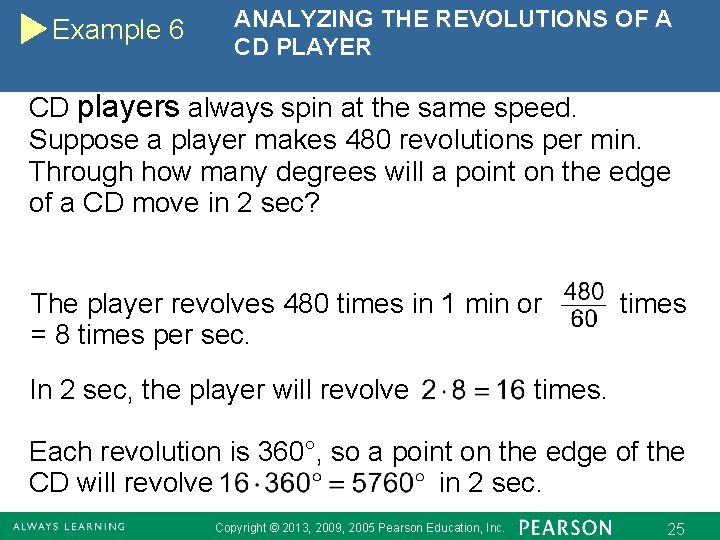# 1 Trigonometric Functions Copyright 2013 2009 2005 Pearson

• Slides: 251 Trigonometric Functions Copyright © 2013, 2009, 2005 Pearson Education, Inc. 1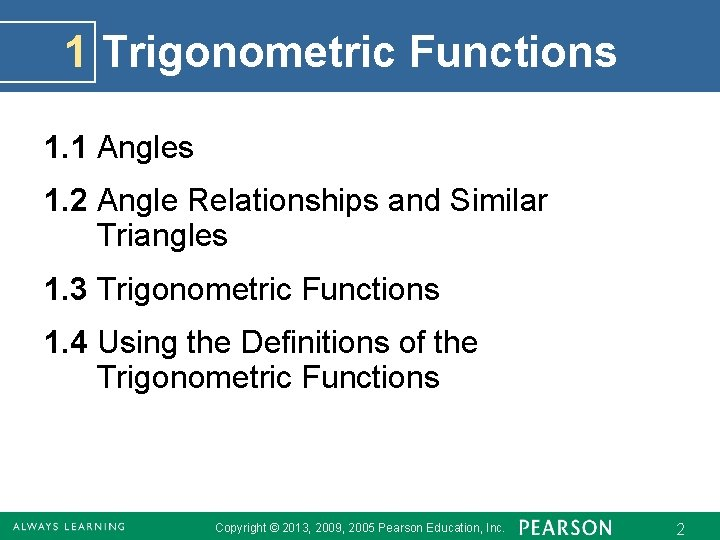1 Trigonometric Functions 1. 1 Angles 1. 2 Angle Relationships and Similar Triangles 1. 3 Trigonometric Functions 1. 4 Using the Definitions of the Trigonometric Functions Copyright © 2013, 2009, 2005 Pearson Education, Inc. 2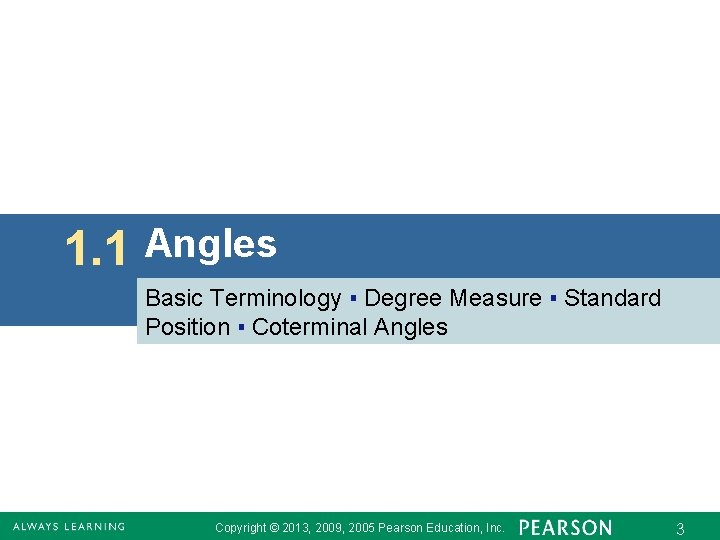1. 1 Angles Basic Terminology ▪ Degree Measure ▪ Standard Position ▪ Coterminal Angles Copyright © 2013, 2009, 2005 Pearson Education, Inc. 3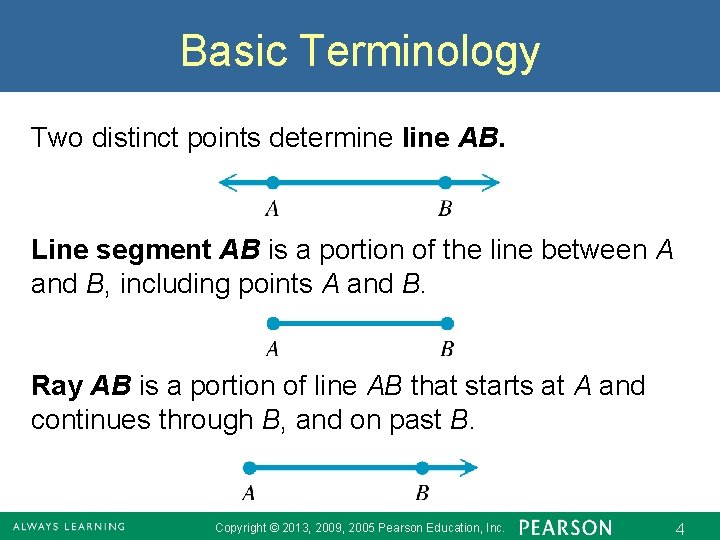Basic Terminology Two distinct points determine line AB. Line segment AB is a portion of the line between A and B, including points A and B. Ray AB is a portion of line AB that starts at A and continues through B, and on past B. Copyright © 2013, 2009, 2005 Pearson Education, Inc. 4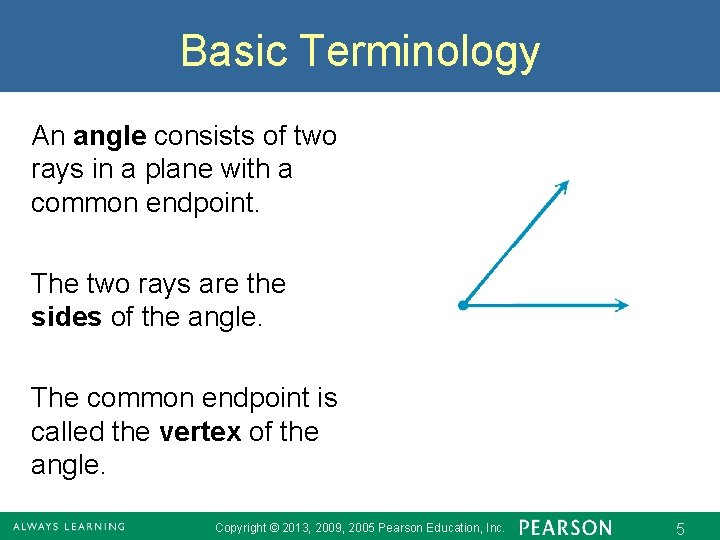Basic Terminology An angle consists of two rays in a plane with a common endpoint. The two rays are the sides of the angle. The common endpoint is called the vertex of the angle. Copyright © 2013, 2009, 2005 Pearson Education, Inc. 5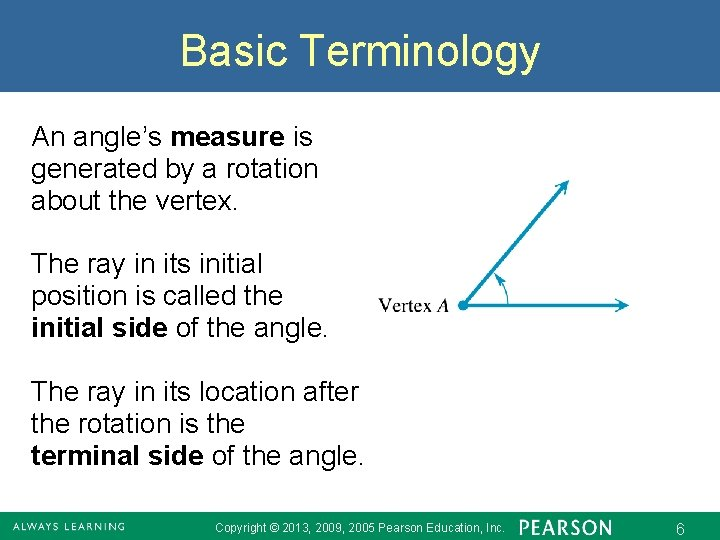Basic Terminology An angle’s measure is generated by a rotation about the vertex. The ray in its initial position is called the initial side of the angle. The ray in its location after the rotation is the terminal side of the angle. Copyright © 2013, 2009, 2005 Pearson Education, Inc. 6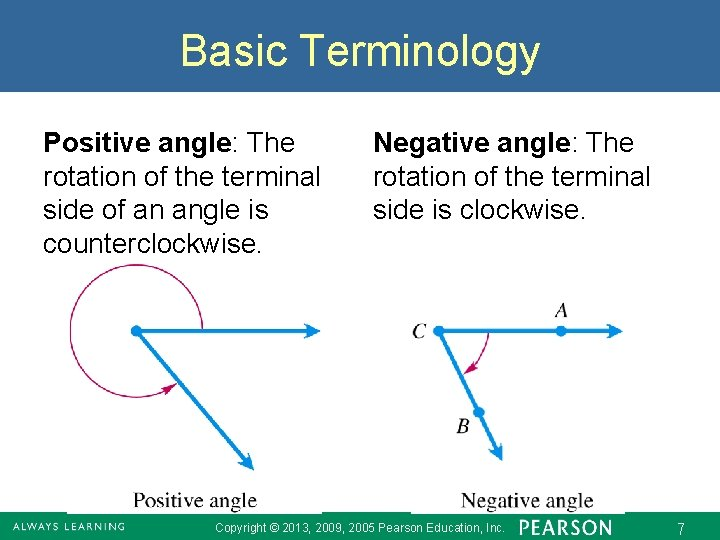Basic Terminology Positive angle: The rotation of the terminal side of an angle is counterclockwise. Negative angle: The rotation of the terminal side is clockwise. Copyright © 2013, 2009, 2005 Pearson Education, Inc. 7Degree Measure The most common unit for measuring angles is the degree. A complete rotation of a ray gives an angle whose measure is 360°. of complete rotation gives an angle whose measure is 1°. Copyright © 2013, 2009, 2005 Pearson Education, Inc. 8Degree Measure Angles are classified by their measures. Copyright © 2013, 2009, 2005 Pearson Education, Inc. 9Example 1 FINDING THE COMPLEMENT AND THE SUPPLEMENT OF AN ANGLE For an angle measuring 40°, find the measure of (a) its complement and (b) its supplement. (a) To find the measure of its complement, subtract the measure of the angle from 90°. Complement of 40° (b) To find the measure of its supplement, subtract the measure of the angle from 180°. Supplement of 40° Copyright © 2013, 2009, 2005 Pearson Education, Inc. 10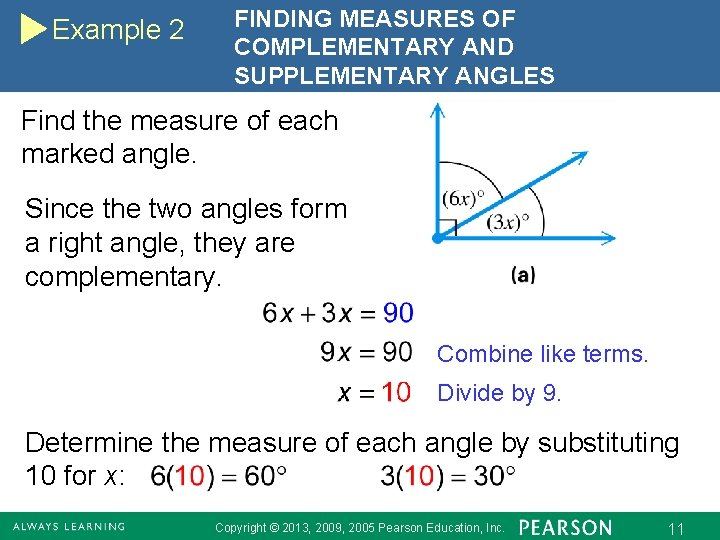Example 2 FINDING MEASURES OF COMPLEMENTARY AND SUPPLEMENTARY ANGLES Find the measure of each marked angle. Since the two angles form a right angle, they are complementary. Combine like terms. Divide by 9. Determine the measure of each angle by substituting 10 for x: Copyright © 2013, 2009, 2005 Pearson Education, Inc. 11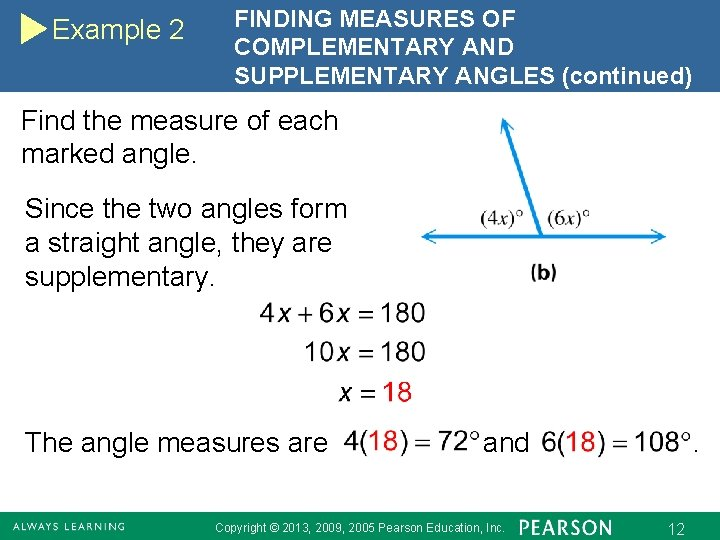Example 2 FINDING MEASURES OF COMPLEMENTARY AND SUPPLEMENTARY ANGLES (continued) Find the measure of each marked angle. Since the two angles form a straight angle, they are supplementary. The angle measures are and Copyright © 2013, 2009, 2005 Pearson Education, Inc. . 12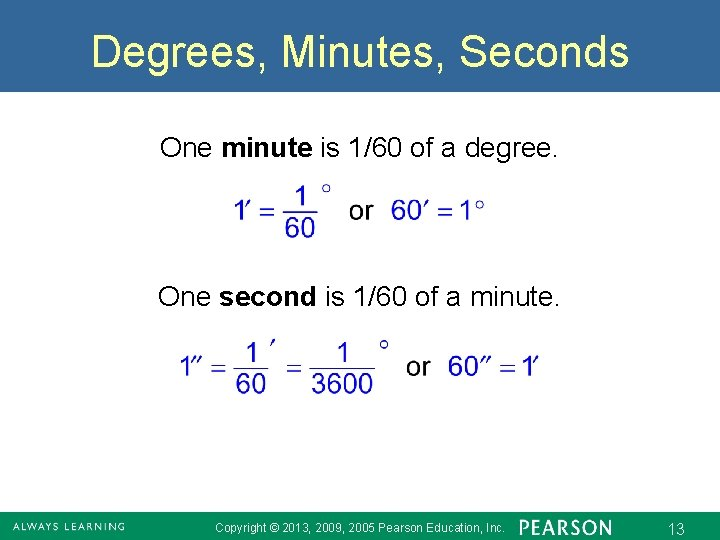Degrees, Minutes, Seconds One minute is 1/60 of a degree. One second is 1/60 of a minute. Copyright © 2013, 2009, 2005 Pearson Education, Inc. 13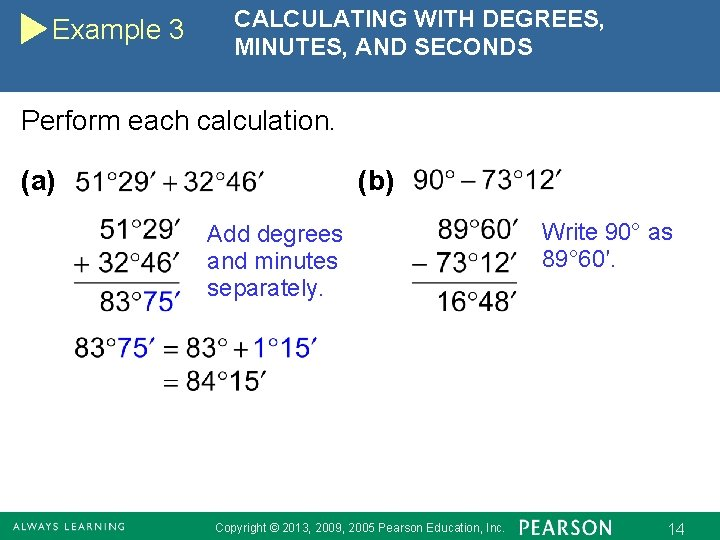Example 3 CALCULATING WITH DEGREES, MINUTES, AND SECONDS Perform each calculation. (a) (b) Add degrees and minutes separately. Copyright © 2013, 2009, 2005 Pearson Education, Inc. Write 90° as 89° 60′. 14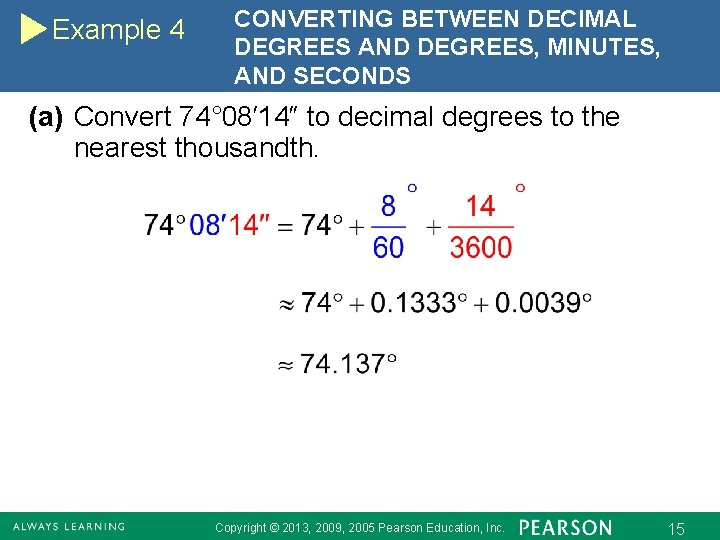Example 4 CONVERTING BETWEEN DECIMAL DEGREES AND DEGREES, MINUTES, AND SECONDS (a) Convert 74° 08′ 14″ to decimal degrees to the nearest thousandth. Copyright © 2013, 2009, 2005 Pearson Education, Inc. 15Example 4 CONVERTING BETWEEN DECIMAL DEGREES AND DEGREES, MINUTES, AND SECONDS (continued) (b) Convert 34. 817° to degrees, minutes, and seconds. Approximate to the nearest second. Copyright © 2013, 2009, 2005 Pearson Education, Inc. 16Standard Position An angle is in standard position if its vertex is at the origin and its initial side lies along the positive x-axis. Copyright © 2013, 2009, 2005 Pearson Education, Inc. 17Quadrantal Angles in standard position whose terminal sides lie along the x-axis or y-axis, such as angles with measures 90 , 180 , 270 , and so on, are called quadrantal angles. Copyright © 2013, 2009, 2005 Pearson Education, Inc. 18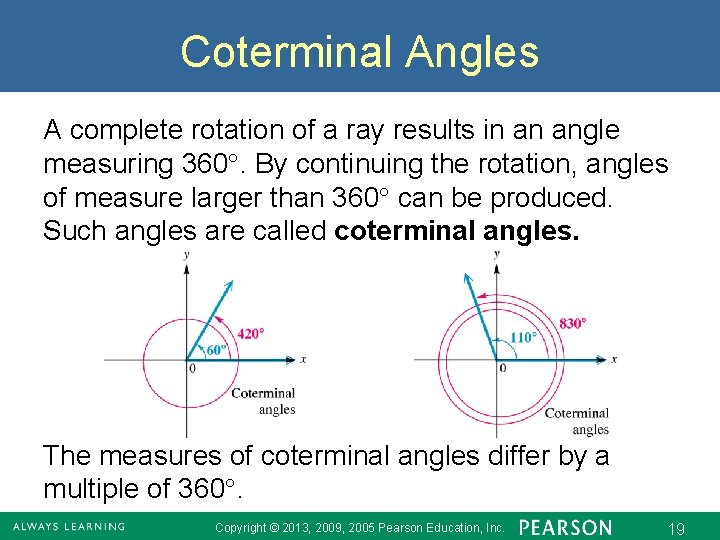Coterminal Angles A complete rotation of a ray results in an angle measuring 360. By continuing the rotation, angles of measure larger than 360 can be produced. Such angles are called coterminal angles. The measures of coterminal angles differ by a multiple of 360. Copyright © 2013, 2009, 2005 Pearson Education, Inc. 19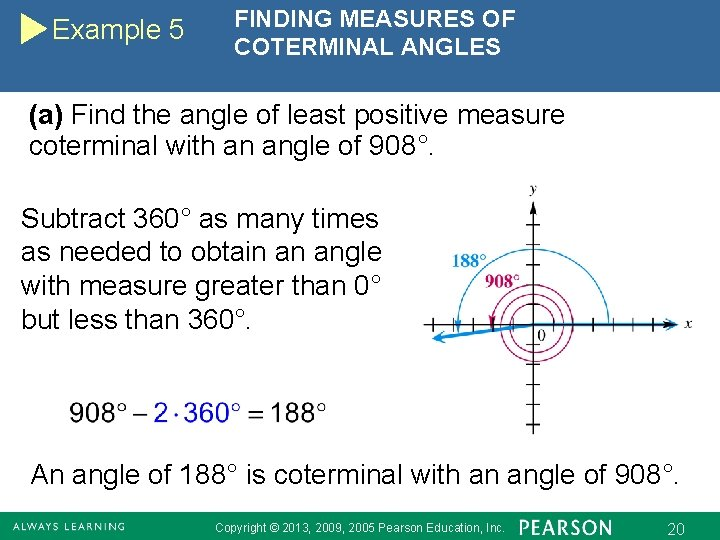Example 5 FINDING MEASURES OF COTERMINAL ANGLES (a) Find the angle of least positive measure coterminal with an angle of 908°. Subtract 360° as many times as needed to obtain an angle with measure greater than 0° but less than 360°. An angle of 188° is coterminal with an angle of 908°. Copyright © 2013, 2009, 2005 Pearson Education, Inc. 20Example 5 FINDING MEASURES OF COTERMINAL ANGLES (continued) (b) Find the angle of least positive measure coterminal with an angle of – 75°. An angle of – 75 °is coterminal with an angle of 285°. Copyright © 2013, 2009, 2005 Pearson Education, Inc. 21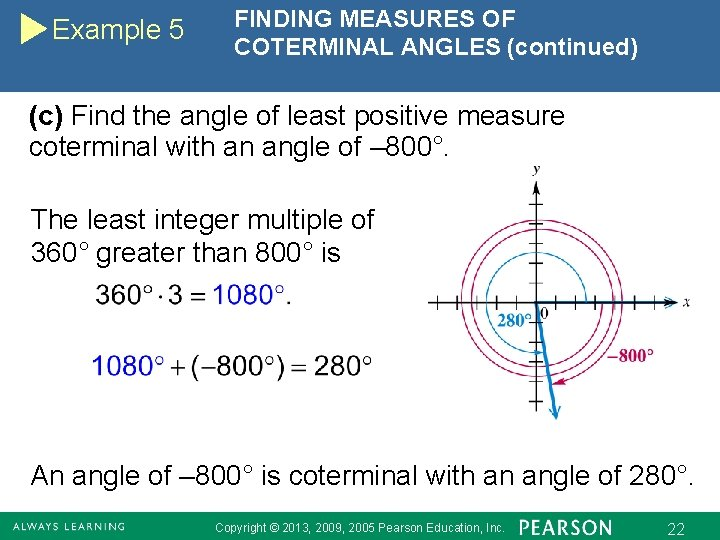Example 5 FINDING MEASURES OF COTERMINAL ANGLES (continued) (c) Find the angle of least positive measure coterminal with an angle of – 800°. The least integer multiple of 360° greater than 800° is An angle of – 800° is coterminal with an angle of 280°. Copyright © 2013, 2009, 2005 Pearson Education, Inc. 22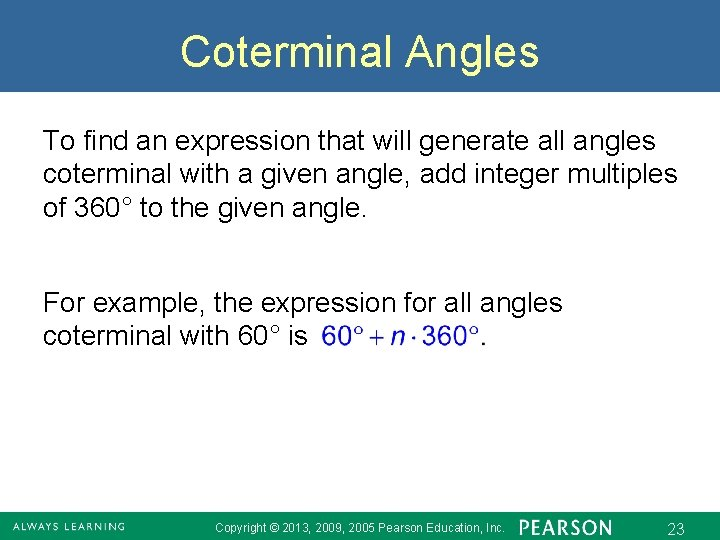Coterminal Angles To find an expression that will generate all angles coterminal with a given angle, add integer multiples of 360° to the given angle. For example, the expression for all angles coterminal with 60° is Copyright © 2013, 2009, 2005 Pearson Education, Inc. 23